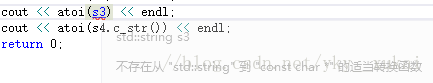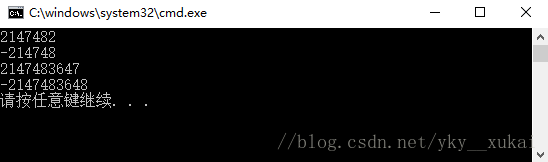# The usage and difference of atoi() and stoi() functions in C + +

Similarities:
The C++ character processing function, the numeric string into an int output
Difference:
Atoi () is const char*, so we must call c_str() to const char* for a string STR. Stoi () is const string*, const char* is not required.
As shown in figure:Stoi () will do the scope check, the default scope is within the scope of int, if beyond the scope will be runtime error!
As shown in figure:Atoi () will not check the range. If it exceeds the range, it will output the upper limit, and if it exceeds the lower limit, it will output the lower limit.
Code:

` `
`//myfirst.cpp--displays a message ` `#include "stdafx.h" ` `#include < iostream> ` `#include < set> ` `#include < string> ` `using namespace std; ` `int main()` `{` `string s1 = "2147482", s2 = "-214748"; ` `string s3 = "214748666666663", s4 = "-21474836488"; ` `cout < < stoi(s1) < < endl; ` `cout < < stoi(s2) < < endl; ` `cout < < atoi(s3.c_str()) < < endl; ` `cout < < atoi(s4.c_str()) < < endl; ` `return 0; ` `}`

Console: